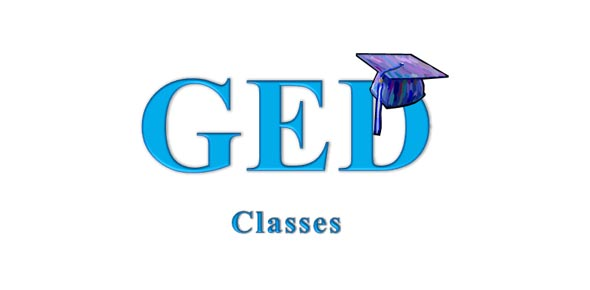# GED Math Quiz

20 Questions | Total Attempts: 3453SettingsThis quiz is to help those taking the GED and needing help in math.

Related Topics
• 1.
Thank you for taking this quiz.  How do you think you did?
• 2.
Solve the following equation for A: 2A/3 = 8 + 4A
• A.

2.4

• B.

1.2

• C.

3.6

• D.

-2.4

• E.

-3.6

• 3.
Lillian wants to invest \$4,000 at 6% simple interest rate for 5 years. How much interest will she receive?
• A.

\$1200

• B.

\$600

• C.

\$960

• D.

\$720

• E.

\$1520

• 4.
If 8x + 5x + 2x + 4x = 114, the 5x + 3 =
• A.

11

• B.

26

• C.

33

• D.

47

• E.

86

• 5.
Which of the following is not a rational number?
• A.

.5

• B.

1/3

• C.

2

• D.

Square Root of 2

• E.

-1

• 6.
What is the absolute value of -4?
• A.

-4

• B.

4

• C.

0

• D.

-2

• E.

2

• 7.
What is the median of the following list of numbers? 4, 5, 7, 9, 10, 12
• A.

8

• B.

7

• C.

9

• D.

7.9

• E.

6

• 8.
-56+8=
• A.

64

• B.

-64

• C.

-49

• D.

49

• E.

-48

• 9.
A nurse has to record her temperatures in Celsius but her thermometer reads Fahrenheit. A patient's temperature is 100.7° F. What is the temperature in °C?
• A.

40.1

• B.

38.2

• C.

35.6

• D.

212.2

• E.

253.2

• 10.
Which of the following correctly identifies 4 consecutive odd integers where the sum of the middle two integers is equal to 28?
• A.

11, 13, 15, 17

• B.

13, 15, 17, 19

• C.

15, 13, 19, 21

• D.

9, 11, 13, 15

• E.

7, 9, 11, 13

• 11.
104 is not equal to which of the following?
• A.

10,000

• B.

10 x 10 x 10 x 10

• C.

100,000

• 12.
Which of the following is not a fraction equivalent to 3/4?
• A.

12/18

• B.

12/16

• C.

27/36

• D.

21/28

• E.

9/12

• 13.
Solve: 4 - (-5 +8) =
• A.

0

• B.

1

• C.

7

• D.

3

• E.

17

• 14.
If Mark purchased x t-shirts at \$6 apiece, and y sweaters at \$20 apiece, which expression represents the total value of the purchases?
• A.

20x + 6y

• B.

3x + 6y

• C.

20x + 5y

• D.

6x + 5y

• E.

6x + 20y

• 15.
Simplify: 8x + 19x - 6y - (-y)
• A.

27x - 7y

• B.

27x -5y

• C.

27x - 18y

• D.

11x - 5y

• E.

11x + 7y

• 16.
If Monique buys 6 dresses at 15% off the normal price x, which expression represents how much she has spent?
• A.

.15(6x)

• B.

15(6x)

• C.

1.5(.6x)

• D.

.15(.6x)

• E.

.15x(6x)

• 17.
(3x + 9)/2 = 9
• A.

9

• B.

5

• C.

3

• D.

10

• E.

4

• 18.
What is the value of 20x - 3y2 if x = 2 and y = 3?
• A.

17

• B.

15

• C.

41

• D.

27

• E.

13

• 19.
Employees of a discount appliance store receive an additional 20% off of the lowest price on an item. If an employee purchases a dishwasher during a 15% off sale, how much will he pay if the dishwasher originally cost \$450?
• A.

\$280.99

• B.

\$256

• C.

\$292.51

• D.

\$306

• E.

\$333.89

• 20.
Amy has 16 jellybeans in her pocket. She has 4 orange ones, 8 purple ones, and 4 blue ones. What is the minimum number of jellybeans she must take out of her pocket to ensure that she has one of each color?
• A.

4

• B.

8

• C.

12

• D.

13

• E.

16